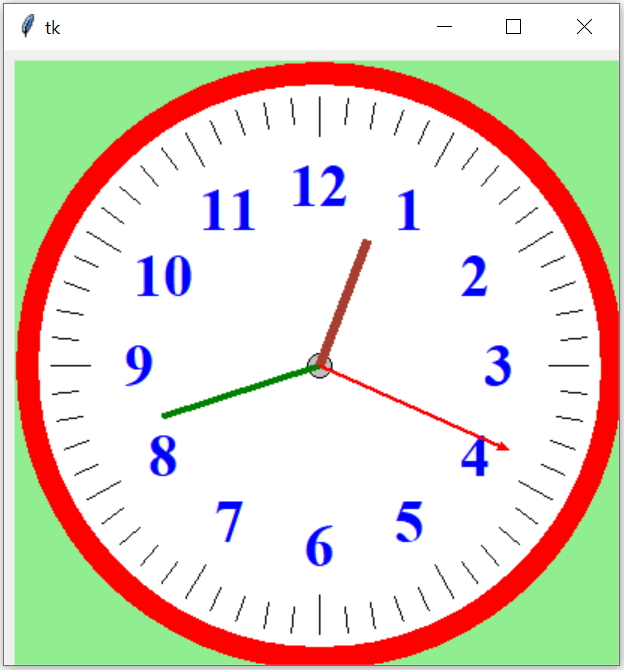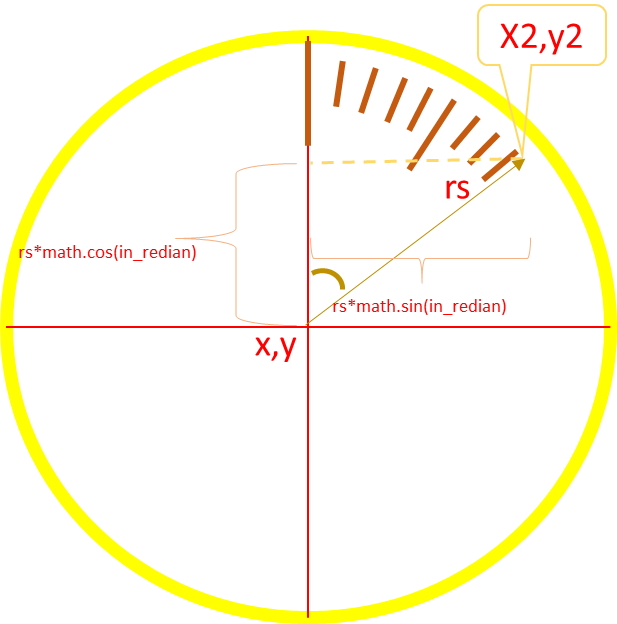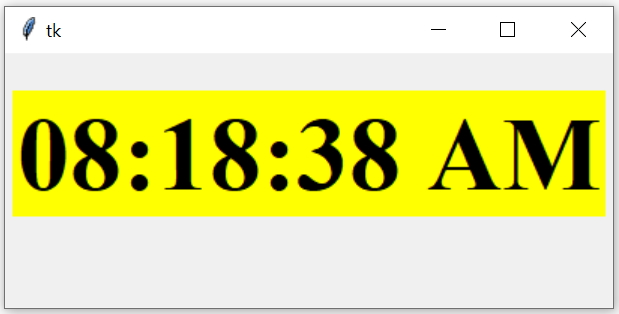# Analog Clock Dial with needlesOnce the dial is ready with all markings ( read part 1 , creating dial ), to this we will add needles to the clock.

Analog clock in Tkinter Canvas with three needles hour, minute, second initializing with local time

Following points may be noted.
• Each segment is 6 degree and there are 60 segments in our dial.
• Each needle has to increase by 6 degree to cross one segment.
• For each 360 degree rotation of second needle, minute needle will move by one segment ( 6 degree ).
• Hour needle moves 30 degree or 5 segments of 6 degree each on every completion of one rotation of 360 degree by minutes needle.
• Zero degree is the 12 O’clock position.
We will use three functions my_second(),my_minute() and my_hour() to display and rotate needles. Using timer we will call ( recursive call ) to my_second() function and on completion of 360 degree we will trigger my_minute() function and on completion of 360 degree of minute needle we will call my_hour().

## Increment in each call to functions

Second needle has to increase by one segment of 6 degree on each call to my_second() function ( on every second ).
Minute needle has to increase by one segment of 6 degree on each call to my_minute() function. ( on every 60 seconds).
Hour needle has to increase by 0.5 degree on each call to my_hour() function.

## Starting angles of Hour minute and second needles

When the clock starts, we must read the local time and accordingly shift the needles ( starting point). From this point we can start moving the needles. Here is how to read the local time in hour minutes and seconds.
``````in_degree = 0 # for creating dial markings
in_degree_s=int(time.strftime('%S')) *6 # local second
in_degree_m=int(time.strftime('%M'))*6 # local minutes
in_degree_h=int(time.strftime('%I')) * 30 # 12 hour format
print(in_degree_h)``````

## Initializing second needle position based on local time

Our variable in_degree_s will hold the local second value.
``````in_radian = math.radians(in_degree_s)
second=c1.create_line(x,y,x2,y2,fill='red',width=2) # draw the needle``````
Above code will draw the second needle based on the local time second value and the variable in_degree_s will hold the degree based on this local time value.

## Rotating Second needle my_second()

As we have initialized the variable in_degree_s based on the local time value of seconds, we will use these variables as global and create the function my_second()

## Use of timer

We used one timer which calls the same function ( recursive ) my_second after 1000 milliseconds (= 1 second ).
``c1.after(1000,my_second)``
Each call to this function will move the second needle by 6 degree (` in_degree_s=in_degree_s+6 `) The movement of second needle is achieved by deleting the second needle and redrawing it at the new coordinates.``````    c1.delete(second) # delete the needle
x2=x+rs*math.sin(in_radian) # Horizontal coordinate of outer edge

second=c1.create_line(x,y,x2,y2,arrow='last',fill='red',width=2)``````
Full code for movement of Second needle is here .
``````def my_second():
global in_degree_s,second
c1.delete(second) # delete the needle
x2=x+rs*math.sin(in_radian) # Horizontal coordinate of outer edge
second=c1.create_line(x,y,x2,y2,arrow='last',fill='red',width=2)
if(in_degree_s>=360): # one rotattion is over if reached 360
in_degree_s=0 # start from zero angle again
my_minute()  # call the minute needle to move one segment.
in_degree_s=in_degree_s+6 # increment of one segment is 6 degree
c1.after(1000,my_second) # recrusive call after 1000 milliseconds``````

## Minute needle

Each complete rotation of second needle will trigger the my_miute() function. Check the lines in above code( inside my_second() function ).
``````if(in_degree_s>=360): # one rotattion is over if reached 360
in_degree_s=0 # start from zero angle again
my_minute()  # call the minute needle to move one segment.
c1.after(1000,my_second) # recrusive call after 1000 milliseconds``````

## Adjusting minute needle based on initial minutes value

The variable in_degree_m will hold the angle for minutes needle after reading the local time minute value. ( check the starting angles of hour , minute and second needles above )
``````in_radian = math.radians(in_degree_m)
minute=c1.create_line(x,y,x2,y2,width=4,fill='green')``````
Full code for Minute needle is here .
``````def my_minute():
global in_degree_m,minute
in_degree_m=in_degree_m+6 # increment for each segment
c1.delete(minute) # delete the previous needle
x2=x+rm*math.sin(in_radian) # Horizontal coordinate of outer edge
y2=y-rm*math.cos(in_radian) # vertical coordinate of outer dege
minute=c1.create_line(x,y,x2,y2,width=4,fill='green')
my_hour() # calling hour needle to move
if(in_degree_m>=360): # One rotation of 360 degree is over
in_degree_m=0``````

## Adjusting hour needle based on initial minutes value

At the starting, hour needle we have to move fraction of one hour based on the minutes value. When local time is 7.45 , the hour needle has to be 3/4 of 7 hour and 8 hour segments.

Note that for 360 degree rotation of minute ( one hour ) the hour needle moves by 30 degree. To get the initial angle for hour needle we have to multiply minutes angle value like this. Here 30/360 = 0.0833333
``````in_degree_h=in_degree_h+(in_degree_m*0.0833333)
hour=c1.create_line(x,y,x2,y2,width=6,fill='#a83e32')``````
The code for hour needle is here
``````def my_hour():
global in_degree_h,hour
in_degree_h=in_degree_h+0.5 # increment in each step
c1.delete(hour) # deleting hour needle
x2=x+rh*math.sin(in_radian) # Horizontal coordinate for outer edge
hour=c1.create_line(x,y,x2,y2,width=6,fill='#a83e32')
if(in_degree_h>=360):
in_degree_h=0``````
From the main script we have to call my_second() once to start the clock.
``````my_second() # calling to start
my_w.mainloop()``````
variablesDetails
rs,rm,rh:Length of Second needle,minute needle and hour needle
x,yCenter coordinates.
x2,y2Outer edge coordinates of three needles .
in_degreeAngle in degree. Each segment is 6 degree
in_radianInputs to sin() and cos() functions value in radian. Value in degree is converted to radian before using with sin() or cos() functions.
Here is the full code with dial and all needlesSubscribe to our YouTube Channel here

## Subscribe

* indicates required
Subscribe to plus2netplus2net.com